# ELECTRIC POTENTIAL To move a charge that is

• Slides: 22ELECTRIC POTENTIAL To move a charge that is at rest, requires a force to cause it. To stop a charge in motion now, requires a force to pause it. Just as a man may need a push (in matters existential) So moving charges in E fields Is an application of POTENTIALELECTRIC POTENTIAL • Keep in mind gravitation and its similarity, so far, with electrostatics. U = mgh (if near the earth’s surface) (For distances large compared to the earth’s radius) Gravitational potential is the potential energy per unit mass. GP = gh or GMe/r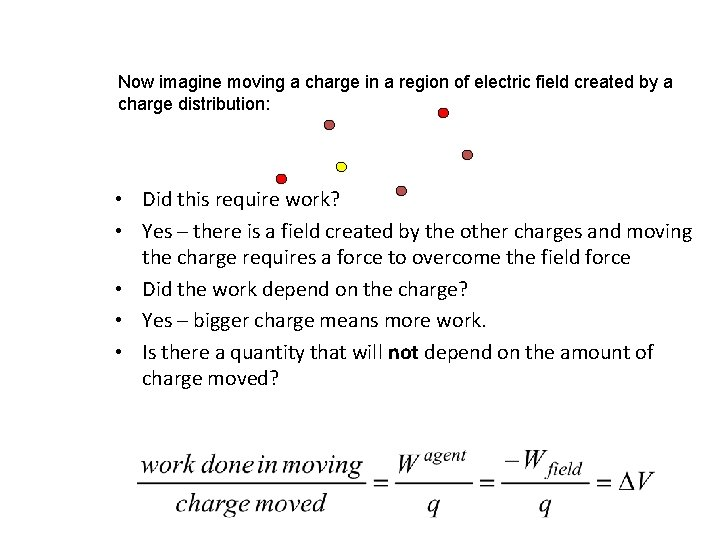Now imagine moving a charge in a region of electric field created by a charge distribution: • Did this require work? • Yes – there is a field created by the other charges and moving the charge requires a force to overcome the field force • Did the work depend on the charge? • Yes – bigger charge means more work. • Is there a quantity that will not depend on the amount of charge moved?ELECTRIC POTENTIAL DIFFERENCE ∆V is called the “electric potential difference” between the two points. Its units are Joules per Coulomb. One Joule per Coulomb is equal to one VOLT The value of the potential difference between two points is determined by the charges that create the electric field, but it does not depend on any charge that might be moved between the two points. Example #1 It takes 3. 2 J to move a 400µC charge between two points separated by 8 m. Calculate the potential difference between the two points.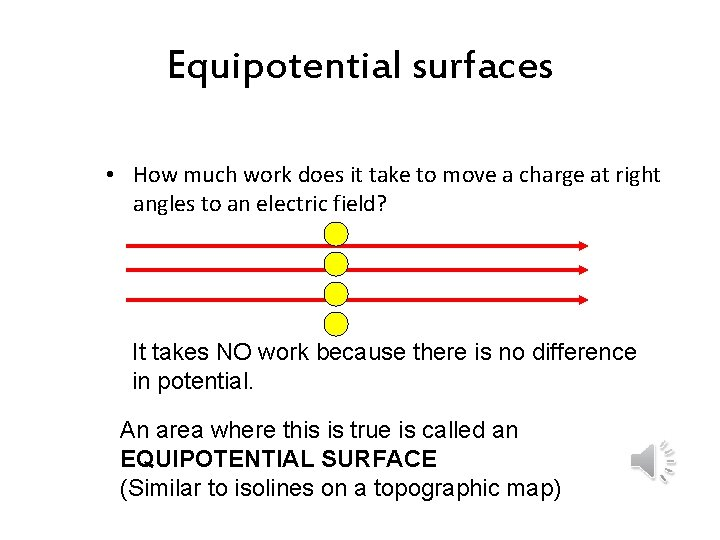Equipotential surfaces • How much work does it take to move a charge at right angles to an electric field? It takes NO work because there is no difference in potential. An area where this is true is called an EQUIPOTENTIAL SURFACE (Similar to isolines on a topographic map)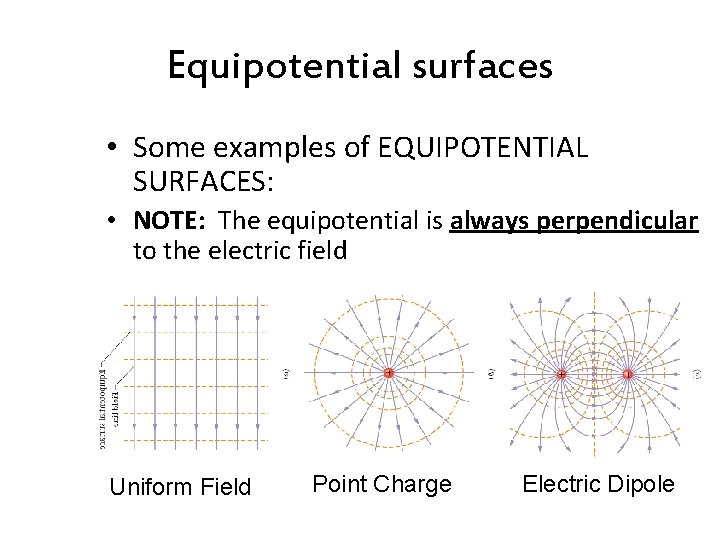Equipotential surfaces • Some examples of EQUIPOTENTIAL SURFACES: • NOTE: The equipotential is always perpendicular to the electric field Uniform Field Point Charge Electric Dipole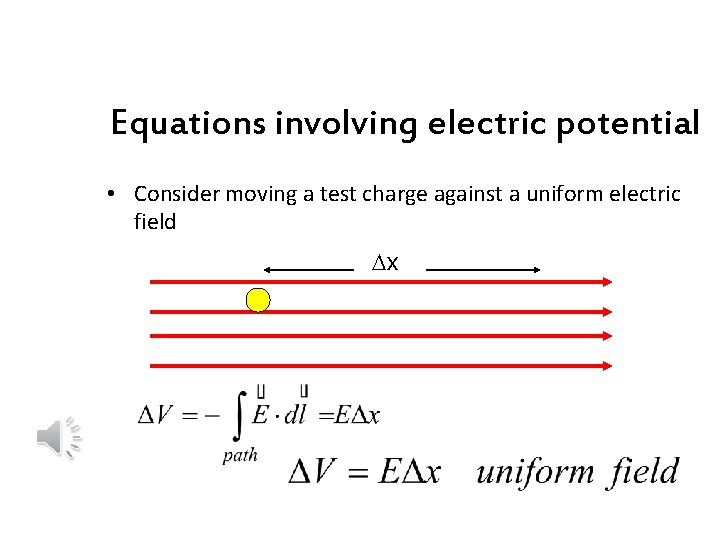Equations involving electric potential • Consider moving a test charge against a uniform electric field Dx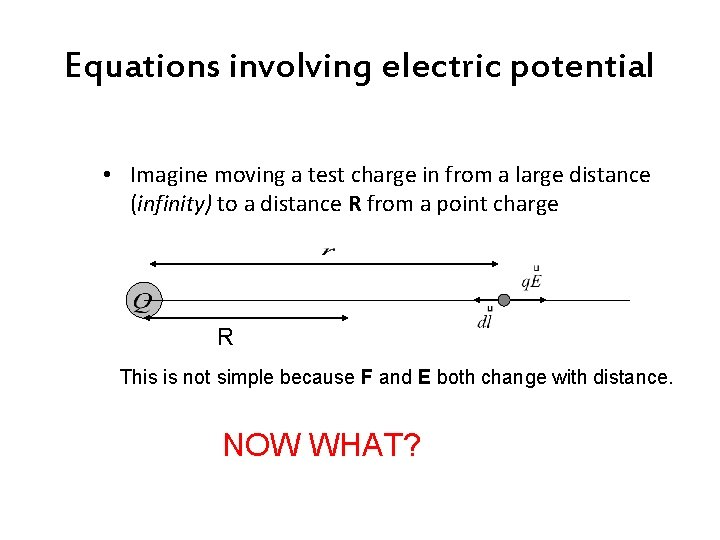Equations involving electric potential • Imagine moving a test charge in from a large distance (infinity) to a distance R from a point charge R This is not simple because F and E both change with distance. NOW WHAT?Equations involving electric potential R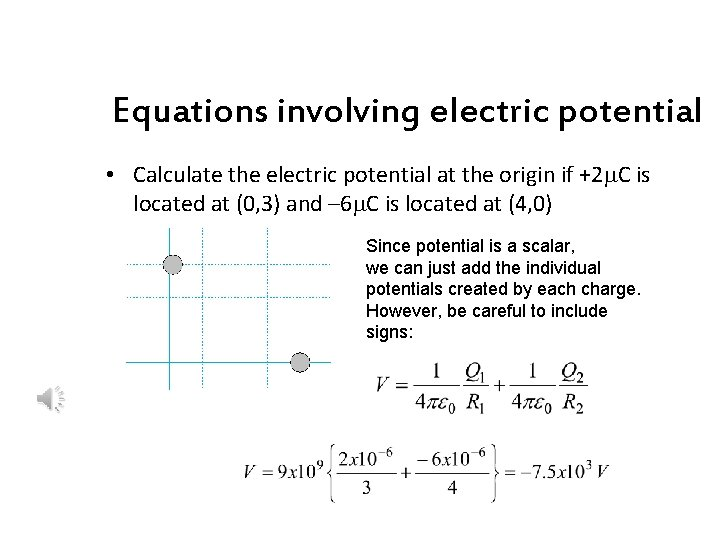Equations involving electric potential • Calculate the electric potential at the origin if +2 m. C is located at (0, 3) and – 6 m. C is located at (4, 0) Since potential is a scalar, we can just add the individual potentials created by each charge. However, be careful to include signs:Equations involving electric potential CONDUCTING SPHERE §Even though the field is zero in the conductor, the potential is not. § The potential has already been chosen as zero at infinity and it takes work to move a charge to the conductor from there. §The potential inside the sphere is equal everywhere to the potential at the surface.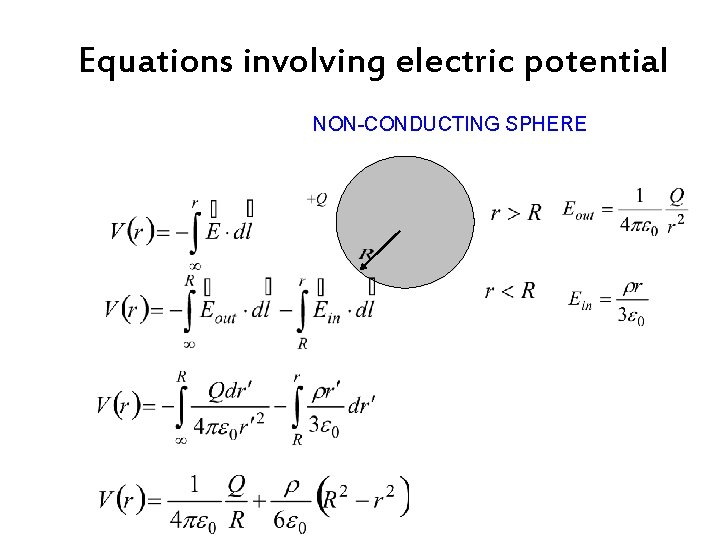Equations involving electric potential NON-CONDUCTING SPHERE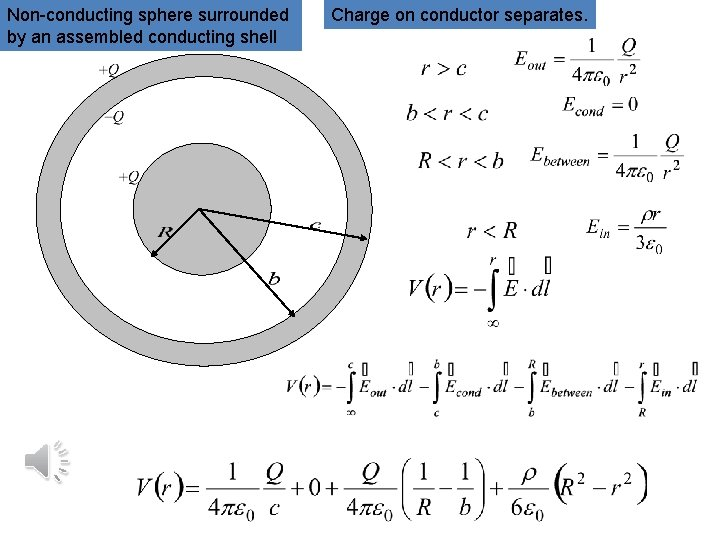Non-conducting sphere surrounded by an assembled conducting shell Charge on conductor separates.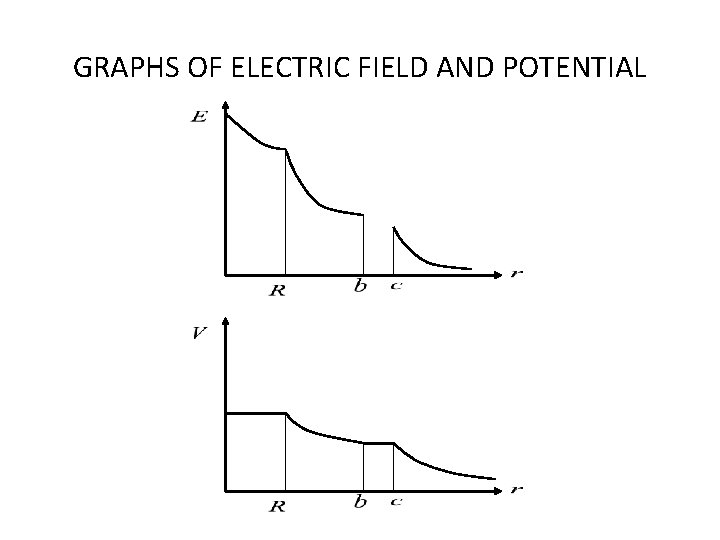GRAPHS OF ELECTRIC FIELD AND POTENTIAL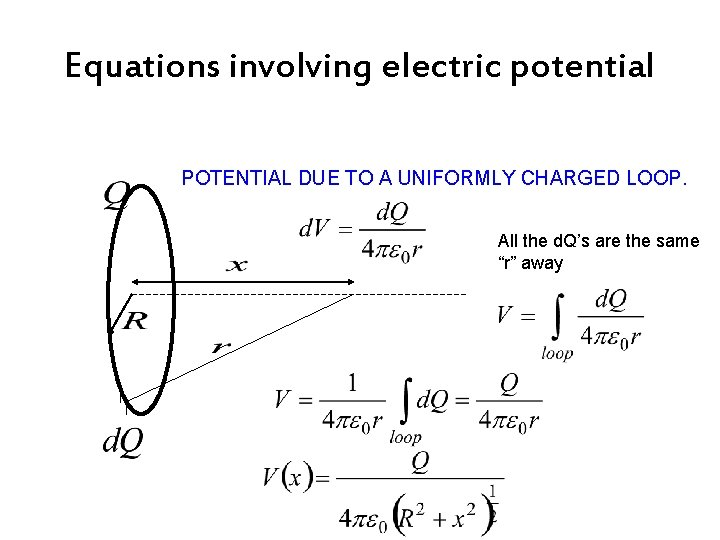Equations involving electric potential POTENTIAL DUE TO A UNIFORMLY CHARGED LOOP. All the d. Q’s are the same “r” away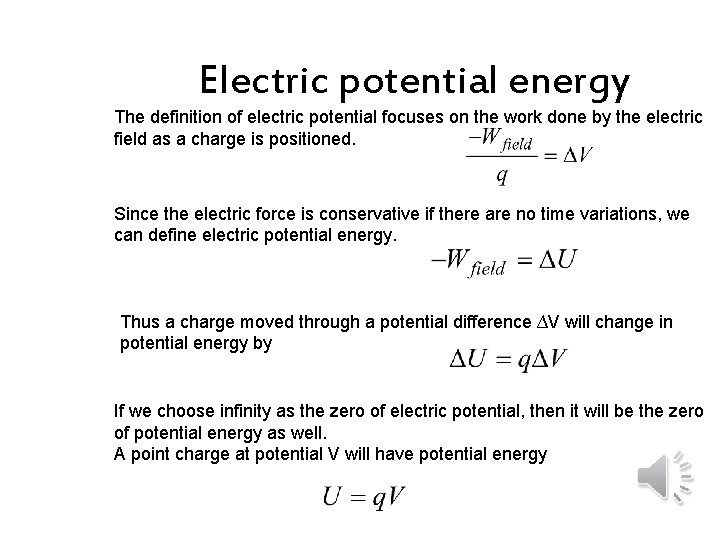Electric potential energy The definition of electric potential focuses on the work done by the electric field as a charge is positioned. Since the electric force is conservative if there are no time variations, we can define electric potential energy. Thus a charge moved through a potential difference ∆V will change in potential energy by If we choose infinity as the zero of electric potential, then it will be the zero of potential energy as well. A point charge at potential V will have potential energy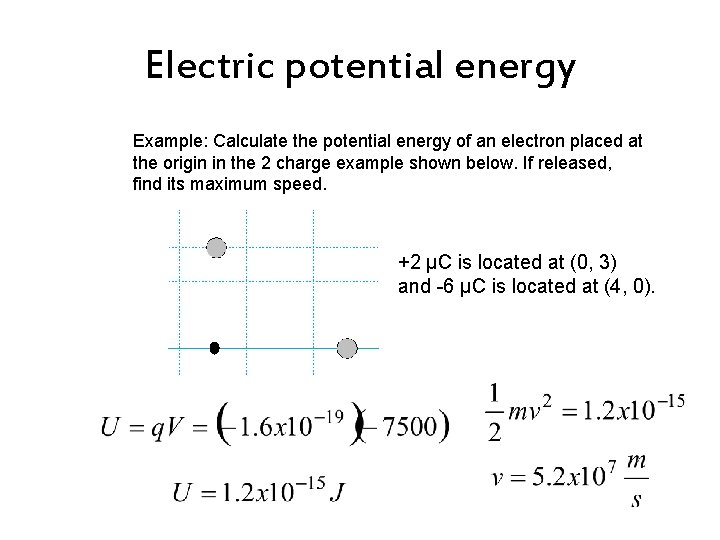Electric potential energy Example: Calculate the potential energy of an electron placed at the origin in the 2 charge example shown below. If released, find its maximum speed. +2 µC is located at (0, 3) and -6 µC is located at (4, 0).The tail of the tape ELECTRIC POTENTIAL ENERGY is: Electric potential • the energy of a charged object in an external electric field. • the energy of the system consisting of the object and the external electric field. • equal to the work done assembling charges. • Equal to the work done moving a charge in an electric field • measured in Joules. • A property of an electric field regardless of whether a charged object has been placed in that field. • Calculated as work per unit charge (V=-W/q) • Commonly referred to as voltage • Symbolized with a V • Measured in Joules per Coulomb or VoltsElectron volt • The amount of energy to move a single elementary charge through a potential difference of exactly one volt. • 1 e. V = (1. 6 x 10 -19 c)(1 v)Where did Ben Franklin sign the Declaration of Independence? At the bottom! That joke had no potential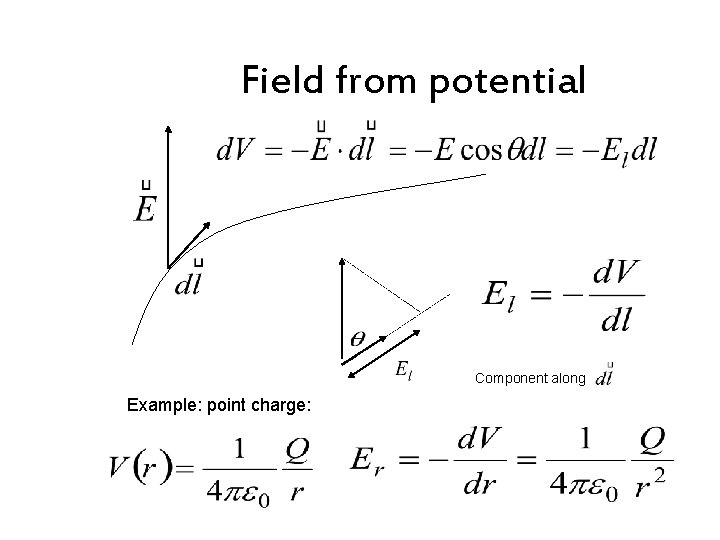Field from potential Component along Example: point charge:Equations involving electric potential ELECTRIC DIPOLE